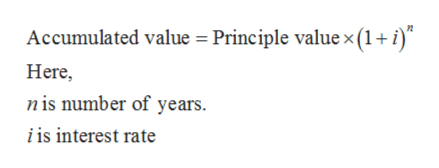# find the interest earned principle 8000 rate .06 compounded annually years 3

Question
15 views

find the interest earned principle 8000 rate .06 compounded annually years 3

check_circle

Step 1

The formula to calculate accumulated value is given below:help_outlineImage TranscriptioncloseAccumulated value = Principle value x (1+ i) Here, nis number of years i is interest rate fullscreen
Step 2

Substitute \$8,000 for principle value, 0.06 for i and 3 for n,

Step 3

The interest earned over three year can be calculated by taking the difference of accum...

### Want to see the full answer?

See Solution

#### Want to see this answer and more?

Solutions are written by subject experts who are available 24/7. Questions are typically answered within 1 hour.*

See Solution
*Response times may vary by subject and question.
Tagged in